# C++ Programs to Reverse Array Element Using Function

In this tutorial you will learn about the C++ Programs to Reverse Array Element Using Function and its application with practical example.

## C++ Programs to Reverse Array Element Using Function

In this tutorial, we will learn to create a C++ program that will Reverse Array Element Using Function using C++ programming.

## Prerequisites

Before starting with this tutorial we assume that you are best aware of the following C++ programming topics:

• Operators in C++ Programming.
• Basic Input and Output function in C++ Programming.
• Basic C++ programming.
• For loop in C++ Programming.
• Functions in C++ Programming.

## Reversing of the array.

The array stores multiple elements in a single variable using the different index values. All the array operations are performed on the index values for manipulation in an array. In this tutorial, we will reverse the elements of the array from start to end.

## Programs to Reverse Array Element Using Function:-

In the program, First, we will initialize the elements of an array. Then we will reverse that array using C++ Programming Language.

## Program:-

To Reverse Array Element Using Function

## Output:-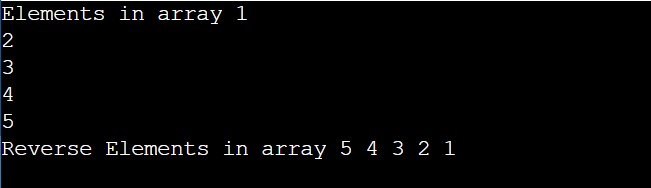In the above program, we have first initialized the required variable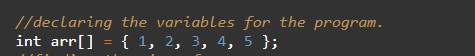• arr = it will hold the elements in an array.
• n = it will hold the number of elements in an array.
• i = it will hold the integer value to control the array.

Printing elements of the array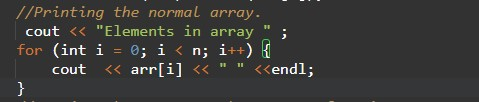Calling of user-defined function in program.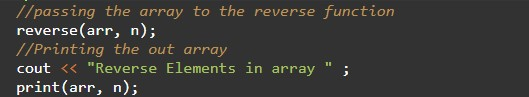Program Code for reverse function.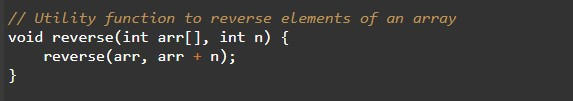Program Code for Print function.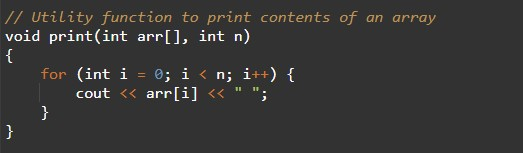In this tutorial we have learn about the C++ Programs to Reverse Array Element Using Function and its application with practical example. I hope you will like this tutorial.